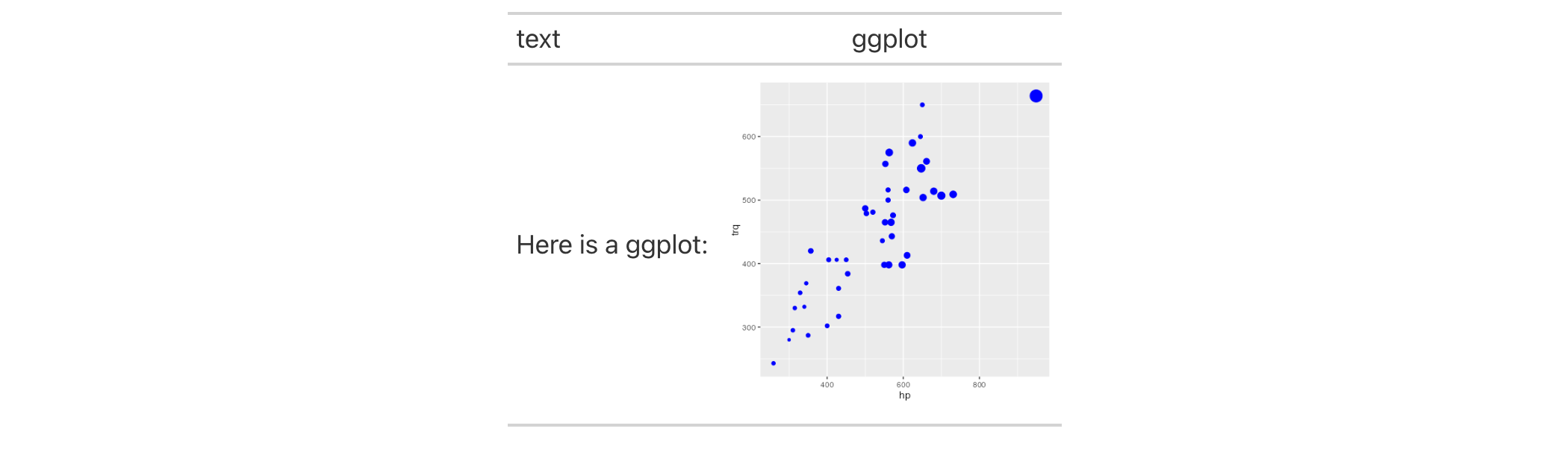We can add a ggplot2 plot inside of a table with the help of the ggplot_image() function. The function provides a convenient way to generate an HTML fragment with a ggplot object. Because this function is currently HTML-based, it is only useful for HTML table output. To use this function inside of data cells, it is recommended that the text_transform() function is used. With that function, we can specify which data cells to target and then include a call to ggplot_image() within the required user-defined function (for the fn argument). If we want to include a plot in other places (e.g., in the header, within footnote text, etc.) we need to use ggplot_image() within the html() helper function.

## Usage

ggplot_image(plot_object, height = 100, aspect_ratio = 1)

## Arguments

plot_object

A ggplot plot object.

height

The absolute height (px) of the image in the table cell.

aspect_ratio

The plot's final aspect ratio. Where the height of the plot is fixed using the height argument, the aspect_ratio will either compress (aspect_ratio < 1.0) or expand (aspect_ratio > 1.0) the plot horizontally. The default value of 1.0 will neither compress nor expand the plot.

## Value

A character object with an HTML fragment that can be placed inside of a cell.

## Details

By itself, the function creates an HTML image tag with an image URI embedded within (a 100 dpi PNG). We can easily experiment with any ggplot2 plot object, and using it within ggplot_image(plot_object = <plot object> evaluates to:

<img src=<data URI> style=\"height:100px;\">

where a height of 100px is a default height chosen to work well within the heights of most table rows. There is the option to modify the aspect ratio of the plot (the default aspect_ratio is 1.0) and this is useful for elongating any given plot to fit better within the table construct.

## Examples

Create a ggplot plot.

library(ggplot2)

plot_object <-
ggplot(
data = gtcars,
aes(x = hp, y = trq, size = msrp)
) +
geom_point(color = "blue") +
theme(legend.position = "none")

Create a tibble that contains two cells (where one is a placeholder for an image), then, create a gt table. Use the text_transform() function to insert the plot using by calling ggplot_object() within the user- defined function.

dplyr::tibble(
text = "Here is a ggplot:",
ggplot = NA
) %>%
gt() %>%
text_transform(
locations = cells_body(columns = ggplot),
fn = function(x) {
plot_object %>%
ggplot_image(height = px(200))
}
)## Function ID

8-3

Other image addition functions: local_image(), test_image(), web_image()